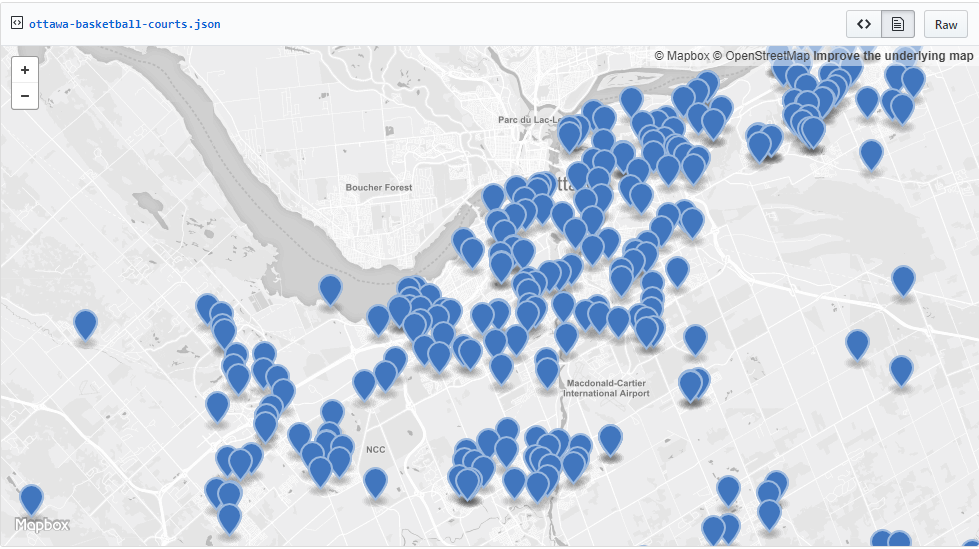Redis GeoSpatial data sets are actually just SortedSets in Redis, there is no secret about it. Basically it provides an easy way to store geo spatial data like longitude/latitude coordinates into Redis.Lets look at some of the commands that Redis provides for Geo Spatial data.

### Redis Geo Datatype – Operations

• GEOADD: Adds or updates one or more members to a Geo Set O(log (N)) where N is the number of elements in the sorted set.
• GEODIST: Return the distance between two members in the geo spatial index represented by the sorted set O(log(N)).
• GEOHASH: Gets valid Geohash strings representing the position of one or more elements from the Geo Sets O(log(N)), where N is the number of elements in the sorted set.
• GEOPOS: Return the longitude,latitude of all the specified members of the geo spatial sorted set at key O(log(N)), where N is the number of elements in the sorted set.
• GEORADIUS: Return the members of a sorted set populated with geo spatial information using GEOADD, which are within the borders of the area specified with the center location and the maximum distance from the center (the radius) O(N+log(M))
• GEORADIUSBYMEMBER: Same as GEORADIUS with the only difference that instead of taking, as the center of the area to query, it takes a longitude and latitude value O(N+log(M))

I wanted to use some open data to showcase the usage of Redis GeoSpatial data in Redis. I chose to use Basketball courts in Ottawa since my son plays a bit of basketball.
Here is a map of basketball courts in Ottawa.